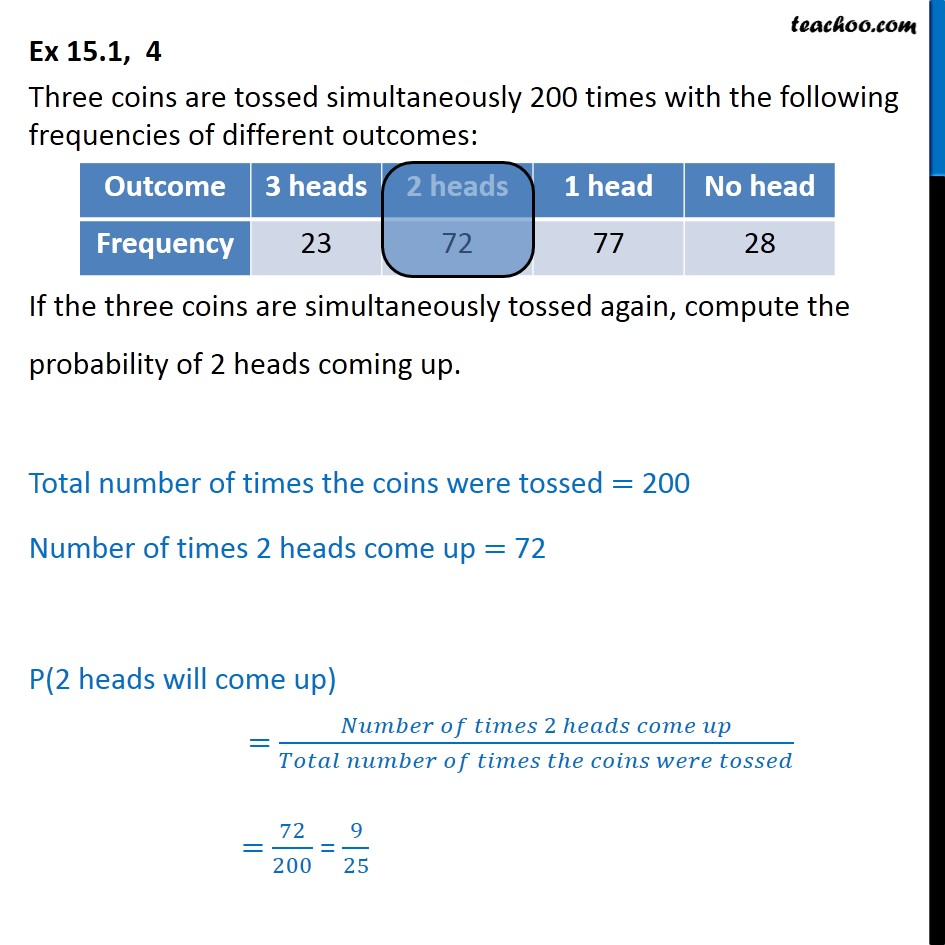Questions - Basic Probability

Probability
Serial order wise
• Questions - Basic Probability

• ExamplesLearn in your speed, with individual attention - Teachoo Maths 1-on-1 Class

### Transcript

Question 4 Three coins are tossed simultaneously 200 times with the following frequencies of different outcomes: If the three coins are simultaneously tossed again, compute the probability of 2 heads coming up. Total number of times the coins were tossed = 200 Number of times 2 heads come up = 72 P(2 heads will come up) = 72/200 = 9/25